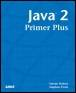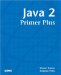# RecursionJava™ 2 Primer PlusBy Steven Haines, Steve Potts Table of Contents Chapter 5.  Methods

Thus far the methods in this chapter have been self-sufficient; they represented a single set of functionality and returned the result of that functionality. Methods are very capable of calling other methods to help solve their problems, but there is another domain of methods that relies on multiple calls to itself. If a method calls itself, that act is referred to as recursion.

Recursive programming can solve some very interesting problems in a very natural and intuitive manner. Consider the factorial example from earlier in the chapter. The result of the factorial operation on a number is the product of that number with all numbers between 1 and the number. The way that that problem was solved in an iterative fashion in Listing 5.2 was to create an intermediate result value equal to the number, and then iterate from that number to 1, multiplying the numbers to the result to get the answer:

` public static int factorial( int n ) {    int result = n;    for( int i=n-1; i>0; i-- ) {      result *= i;    }    return result;  } `

Consider passing the number 4 to this method:

` int result = 4;  for( int i=3; i>0; i-- ) {    result *= i;  }  Or  int result = 4; // result is 4  result *= 3; // result is 12  result *= 2; // result is 24  result *= 1; // result is 24 `

This is a brute force (manually do every computation) way of solving this problem, but there is an elegant, recursive way of solving it. Listing 5.4 shows this recursive solution.

##### Listing 5.4 FactorialRecursive.java
` public class FactorialRecursive {    public static int factorial( int n ) {      if( n == 1 ) return 1;      return n * factorial( n-1 );    }    public static void main( String[] args ) {      System.out.println( "5 factorial = " + factorial( 5 ) );    }  } `

Listing 5.4 defines the factorial method to return 1 if 1 is passed to it (1! = 1), otherwise it returns the product of the number passed to it with the factorial of the number minus 1. The calls for this solution would look as follows:

` factorial( 5 ) = 5 * factorial( 4 )  factorial( 4 ) = 4 * factorial( 3 )  factorial( 3 ) = 3 * factorial( 2 )  factorial( 2 ) = 2 * factorial( 1 )  factorial( 1 ) = 1 `

You will see the following after unwinding the call stack.

` 1 * 2 * 3 * 4 * 5 = 120 `

The key to using recursion is that you must define a stopping point otherwise the recursion will repeat indefinitely. Recursion takes a lot of forethought and planning, but once a solution is found there is usually not too much code and the solution is very elegant.

#### Recursion Versus Iteration

Just looking at the factorial example you can see that both solved the same problem but in quite different ways. I am sure that after you spend some time meditating over the recursive code you will agree that the solution is more elegant.

All recursive problems can be solved through iteration, although not as "pretty," but when would you choose one over the other? From a performance standpoint the answer to this question is obvious after you understand how method calls work (back to the 1s and 0s).

Method calls in Java require the allocation of a section of memory to hold all the method parameters passed to it, plus if the method is not static, a copy of the method executable that will process the invocation. Iterative methods on the other hand, setup the memory for the method call once, and then reference variables in memory for both conditional and increment statements. The end result is that calling methods is far more expensive in terms of memory and CPU time than iteratively checking and incrementing variables in memory.

If performance is of the utmost importance to you, be sure to solve your problems iteratively, not recursively. On the other hand, if you are more concerned with understanding and maintaining your code, you might consider recursive programming.

 TopJava 2 Primer Plus
ISBN: 0672324156
EAN: 2147483647
Year: 2001
Pages: 332

Similar book on Amazon

flylib.com © 2008-2017.
If you may any questions please contact us: flylib@qtcs.net